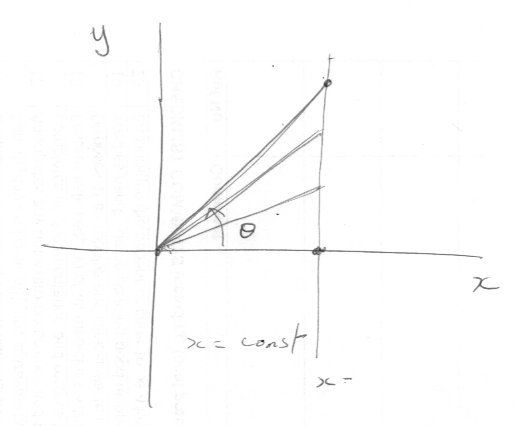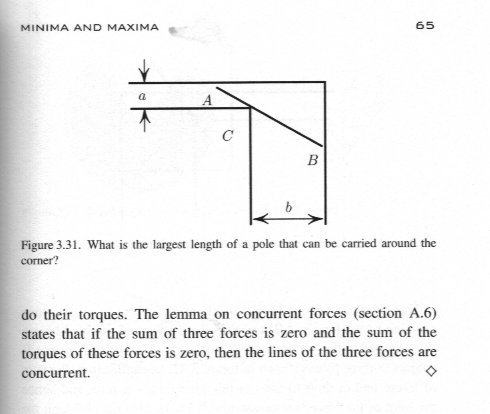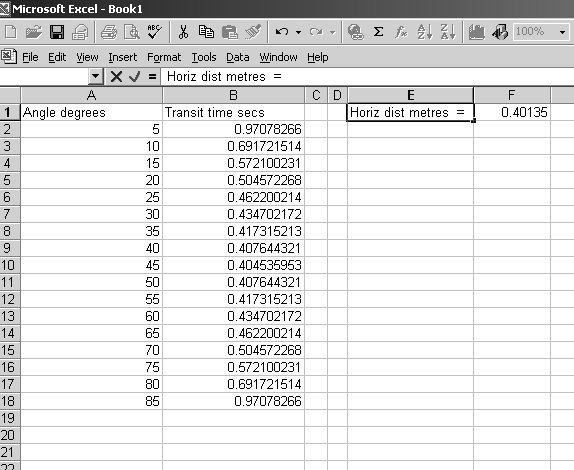# Interesting Inclined Plane Problem

## Recommended Posts

A small block was placed on a smooth inclined plane. The horizontal block distance from the lower end of the incline is b = 40.135 cm. The angle of inclination was adjusted so that the sliding time of the block was as short as possible, each time placing the block so that the distance b did not change. find the shortest possible sliding time T of the block.

Assume that the acceleration due to gravity is g = 9.81 m /s2. Skip friction and air resistance.

Any hints Highly appreciated. I just have a problem with correlating the distance b with the maximum angle possible to make the block even slide from the ramp.

##### Share on other sites

44 minutes ago, Classical Physicist said:

A small block was placed on a smooth inclined plane. The horizontal block distance from the lower end of the incline is b = 40.135 cm. The angle of inclination was adjusted so that the sliding time of the block was as short as possible, each time placing the block so that the distance b did not change. find the shortest possible sliding time T of the block.

Assume that the acceleration due to gravity is g = 9.81 m /s2. Skip friction and air resistance.

Any hints Highly appreciated. I just have a problem with correlating the distance b with the maximum angle possible to make the block even slide from the ramp.

This is known as the brachistochrone problem.

This question is often used to indtroduce the calculus of variations and Euler-Lagrangian mechanics.

##### Share on other sites

Thanks! Had no idea that it's related to the brachistochrone problem. How would you go about Solving this?  Possibly using the "more basic" maths?

##### Share on other sites

I have no idea of your mathematical background, but the link I gave has video and maths solutions galore.

So enjoy and come back if you still have problems.

29 minutes ago, Classical Physicist said:

How would you go about Solving this?  Possibly using the "more basic" maths?

here is a start

Let θ be the angle of inclination of the plane.

Then the vertical distance the block falls  =  ??? (for you to fill in)

Now we pick an equation from kinematics that relates to time and acceleration and equate this to the vertical distance

Edited by studiot
##### Share on other sites

56 minutes ago, studiot said:

Let θ be the angle of inclination of the plane.

Then the vertical distance the block falls  =  ??? (for you to fill in)

So I shall use the basic trig here right?  Assuming inclined plane has a shape of right triangle with base b, hypotenuse c and height x (vertical distance)

We get cos (θ)=x/c ===> cos (θ)*c=x , and now the kinematics… Right?

##### Share on other sites

4 minutes ago, Classical Physicist said:

So I shall use the basic trig here right?  Assuming inclined plane has a shape of right triangle with base b, hypotenuse c and height x (vertical distance)

We get cos (θ)=x/c ===> cos (θ)*c=x , and now the kinematics… Right?

I assume the angle of inclination of the plane is measured from the horizontal. That would be usual.

Please don't use x for vertical distance it confuse people, me in particular.

Let us say that y is the vertical distance. And the horizontal distance is x. The hypotenuse is not needed.

Then the tangent (not the cosine) is needed

tan θ = vertical distance/horizontal distance = y/x

Since x is given yes we now need a kinematic equation, do you know any?

##### Share on other sites

yes!... my concentration right now is very "diffused" if that makes sense- basically I'm trying to do as many problems as I can, without giving too much attention to the details… So Sorry for taking a lot of time to respond, I'll try to limit myself down to this problem right now… hah, I'm seeing you're from UK, so Right now it's quite late (presumably close to midnight ) So I understand you won't be able to respond for some time, so if you don't mind me asking... when will you have some free time so that you'll be active on this forum?##### Share on other sites

I'm still here

What kinematic equations do you know  connecting distance, time and acceleration?

11 minutes ago, Classical Physicist said:

when will you have some free time so that you'll be active on this forum?I'm still here

What kinematic equations do you know  connecting distance, time and acceleration?

##### Share on other sites

Quote

What kinematic equations do you know  connecting distance, time and acceleration?

the basic ones? quite a few
that's the most general an basic one
x(t)= x0+v0xt+(axt2/2)

alternatively since we don't deal with any quadratic function over here :
x(t)= x0 +vt

by the way I am new here and for some reason can't have more than five post for now - I think you'd have to help me get some points or idk, just so that i can post here

Edited by Classical Physicist
edit
##### Share on other sites

Sorry I didn't realise you had just joined.

The 5 post limit is only in the first 24 hours.
This cuts down on spammers.

After that you have (preferably sensible) unlimited posts.

However I think there is a problem with your description of the question.

2 hours ago, Classical Physicist said:

The horizontal block distance from the lower end of the incline is b = 40.135 cm

2 hours ago, Classical Physicist said:

each time placing the block so that the distance b did not change

If this is true there is no solution.

Look carefully at my sketch.If the horizontal distance, x, is always 40.135cm then it is constant.

The line x = a constant is vertical and parallel to the y axis.

So as the inclination, θ, increases, the slope distance (hypotenuse) increases until it is infinite when θ = 90.

ARE YOU QUITE SURE YOU DO NOT MEAN THAT THE HYPOTENUSE DISTANCE IS CONSTANT ?

33 minutes ago, Classical Physicist said:

alternatively since we don't deal with any quadratic function over here :
x(t)= x0 +vt

This one is only for constant velocity and the velocity is not constant here

(That is how you get acceleration)

34 minutes ago, Classical Physicist said:

the basic ones? quite a few
that's the most general an basic one
x(t)= x0+v0xt+(axt2/2)

You do indeed need this one, but the first two terms on the right are zero.

And you will need to differentiate it to get a minimum.

But it is not a difficult process.

But again, please don't use 'x'
You may want to measure in a different direction and x is not general it refers to the horizontal.

The general symbols for 'distance' is usually taken as 's', whcih can be measure up, down, sideways, along a straight or curve or jagged path or whatever.

##### Share on other sites

I owe you a complete apology because I did not read your opening post properly and thereby misunderstood it.

So I charged off in the wrong direction and in particular said there is no solution.

Of course there is a solution.

However the good news is that all was not wasted because my Physics approach was basically correct.

As you said this is an interesting problem. +1 for that

Quote

# Interesting Inclined Plane Problem

It is interesting because the Physics can help the Maths

And the Maths can Help the Physics.

Here is my revised analysis

Going through it,

First we establish the distance travelled down the hypotenuse, h , by simple trigonometry. (Maths)

Then we establish that same distance from kinematic considerations. (Physics)

Then we equate the two (Maths)

Then we substitute values for the acceleration noting that the component of vector quantity a down the slope is given by g sinθ  (Physics)

A bit of mathematical manipulation leads us to the bottom equation for t2. (Maths)

Now the answer of a Physicist who does not want to do calculus might look at this equation and say
"If t2 is a minimum then t is a minimum at the same point or value of θ
4b/g is a constant so the minimum occurs when sin2θ is a maximum
Drawing up a table of values of sin2θ as on the right tells me that this happens at around θ = 40o
Or I can plot these and get a more accurate answer at 45o."                                                                                                 (Physics)

Our Mathematician on the other hand may well differentiate the equation to find that same minimum at dt/dθ or d(t2)/dθ.  (Maths)

We can plough through that maths if you like.

This is not quite the brachistochrone problem since the curve is given as a straight line and so the acceleration is constant and we can use a simple kinematic equation.

Finally since you like this sort of thing, have a look at the book by Mark Levi

Here is an extract from his chapter on maxima and minima##### Share on other sites

9 hours ago, studiot said:

I owe you a complete apology because I did not read your opening post properly and thereby misunderstood it.

I owe you a lot for the fact that You are willing to help me understand it, by a TON! I am extremely grateful for it.

So I took a look at your notes here, and As I understand the ideal θ will be equal to θ=45o,   because sin(2*45o)= 1, so the best possible value that can be in the denominator,

Plugging those values in, I got these results:

9 hours ago, studiot said:

Now the answer of a Physicist who does not want to do calculus might look at this equation and say

"If t2 is a minimum then t is a minimum at the same point or value of θ
4b/g is a constant so the minimum occurs when sin2θ is a maximum
Drawing up a table of values of sin2θ as on the right tells me that this happens at around θ = 40o
Or I can plot these and get a more accurate answer at 45o."                                                                                                 (Physics)

Our Mathematician on the other hand may well differentiate the equation to find that same minimum at dt/dθ or d(t2)/dθ.  (Maths)

We can plough through that maths if you like.

Sure as Hell I would, because I don't get the kind of differentiation we can do here, - especially here with the respect to certain variables, I see you went for differentiating with respect to the angle --- dθ, and so the left hand side sure is d(t2)/dθ , but right side is simply the 4b/g *1/sin(2θ) - so it would get messy, (correct me if I'm wrong)

9 hours ago, studiot said:

Finally since you like this sort of thing, have a look at the book by Mark Levi

Here is an extract from his chapter on maxima and minima

Also this leaves me wondering, are you referring to the 3.30 problem or 3.31 as in the figure above?  By reading it- I get even more confused, because why on earth would you want to depict the distance by a moving mechanism of a piston? - Sorry for being rough but it's quite funny way to measure the distance.

I just don't understand how it is related to finding the shortest possible time? sorry for "being ignorant", but I'm trying as much as I can.Edited by Classical Physicist
e
##### Share on other sites

Hey, I am awaiting your response!##### Share on other sites

1 hour ago, Classical Physicist said:

Hey, I am awaiting your response!Here is an excel spreadsheet at 5o intervals17 hours ago, Classical Physicist said:

Sure as Hell I would, because I don't get the kind of differentiation we can do here, - especially here with the respect to certain variables, I see you went for differentiating with respect to the angle --- dθ, and so the left hand side sure is d(t2)/dθ , but right side is simply the 4b/g *1/sin(2θ) - so it would get messy, (correct me if I'm wrong)

Yes it gets a bit messy but not too much.

Did you understand the use of the double angle formula?

sin 2θ = 2sinθcosθ

17 hours ago, Classical Physicist said:

Also this leaves me wondering, are you referring to the 3.30 problem or 3.31 as in the figure above?  By reading it- I get even more confused, because why on earth would you want to depict the distance by a moving mechanism of a piston? - Sorry for being rough but it's quite funny way to measure the distance.

I just don't understand how it is related to finding the shortest possible time? sorry for "being ignorant", but I'm trying as much as I can

I was recommending the book to you as you can see from its fron cover, Levi sets out to do physics instead of maths and develops some fascinating and  ingenious ways of connecting well known Maths theorems to the physical world.

I though you would be interested.

The only part of the extract is the picture moving the pole round the corner.
The rest of the text is not relevant.

To return it to xls, remove the final .txt and you can play with other values

The base distance, b, is input into box F1 so it can be changed for other distances.

Edited by studiot
##### Share on other sites

1 hour ago, studiot said:

Here is an excel spreadsheet at 5o intervals

Yes it gets a bit messy but not too much.

Did you understand the use of the double angle formula?

sin 2θ = 2sinθcosθ

I was recommending the book to you as you can see from its fron cover, Levi sets out to do physics instead of maths and develops some fascinating and  ingenious ways of connecting well known Maths theorems to the physical world.

I though you would be interested.

The only part of the extract is the picture moving the pole round the corner.
The rest of the text is not relevant.

To return it to xls, remove the final .txt and you can play with other values

The base distance, b, is input into box F1 so it can be changed for other distances.

Huge thanks for making a spreadsheet, - so now for sure  the sin(45) gives the answer I was looking for.  And yeah, I understood the use of double angle formula there, too - a smart way to use it in these type of problems, and I also got the book, and Will certainly have a look, ...explaining calculus problems with some physics sounds very promising!

I have some other quite "extraordinary" problems to go through, would you also like to take a look at them?##### Share on other sites

58 minutes ago, Classical Physicist said:

Huge thanks for making a spreadsheet, - so now for sure  the sin(45) gives the answer I was looking for.  And yeah, I understood the use of double angle formula there, too - a smart way to use it in these type of problems, and I also got the book, and Will certainly have a look, ...explaining calculus problems with some physics sounds very promising!

I have some other quite "extraordinary" problems to go through, would you also like to take a look at them?So here is one way to differentiate

The rule for complicated tig expressions is "First the power, then the trig, then the angle"

note this is  the sine function raised to a power,  not the use of sin-1 which means the inverse sine or arcsin.

So taking the square root and separating the square root of the constant we have

$t = \sqrt {\frac{{4b}}{g}} *\sqrt {\frac{1}{{\sin 2\theta }}} = \sqrt {\frac{{4b}}{g}} *{\left( {\sin 2\theta } \right)^{ - \frac{1}{2}}}$

using my rule above we can differentiate directly

$\frac{{dt}}{{d\theta }} = \sqrt {\frac{{4b}}{g}} *\frac{{ - 1}}{2}*{\left( {\sin 2\theta } \right)^{ - \frac{3}{2}}}*\left( {\cos 2\theta } \right)*2$

Other ways involve using the reciprocals of the trig functions so 1/sin2θ = cosec2θ

But we eventually end up with the same product.

So looking at our derivative and setting it to zero this occurs when

sin2θ = 0o or 90o

or when cos2θ = 90o

as the only values in range.

Yes wheel out your extra ordinary problems, but one at a time please.

A final question,

Do you understand significant figures?

We only know g to 3 s.f.

So there is no point recording more than 4 sf in the calculations for the values of other quantities.

Edited by studiot
##### Share on other sites

21 minutes ago, studiot said:

Yes wheel out your extra ordinary problems, but one at a time please.

A final question,

Do you understand significant figures?

We only know g to 3 s.f.

So there is no point recording more than 4 sf in the calculations for the values of other quantities.

I'm so grateful, for your help, I really get to understand the physics on so much deeper level now as the time goes on, I'll post some more problems in new threads, but as You said -one at a time! Also yeah I Get the significant figures. - so for

T=0.40452441211872491886336935537142 s

we simply get t=0.405s ,:)

##### Share on other sites

for  now I'll have my physics problems posted in "homework help" - because the moderators don't like threads like these in this subtopic

## Create an account

Register a new account